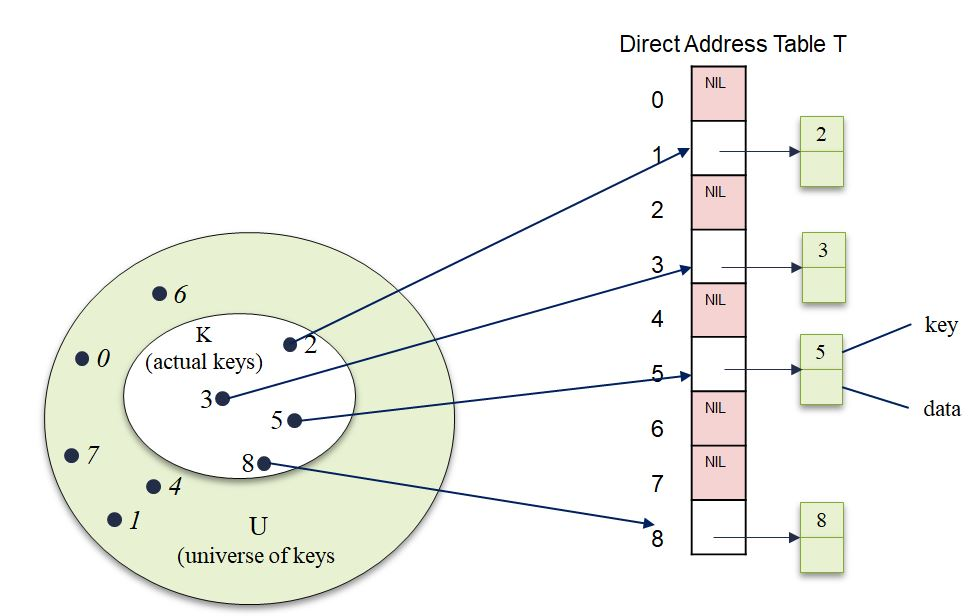# Introduction to Hash Tables and Direct Addressing

We would cover the following:

1. Introduction to Hash Tables

A hash table is a data structure used for implementing dictionaries which support dictionary operations such as INSERT, SEARCH and DELETE. Such a data structure stored data in key-value pairs where each key is associated with a particular value.

A typical application is in the compiler symbol table used during compilation. Here the keys corresponds to the identifiers (variables) used in the program  which has corresponding values. In the worst case, searching for an element in a has table has the same performance as searching for an element in a  linked list which is Θ(n). However, hash tables can achieve a constant time O(n) search performance on the average.

2. Array vs Hash Tables

A hash table generalizes the notion of arrays where the elements can be accessed using the index of the particular element. This is exactly the case when the number of keys to store is the same as the number of positions available. In hash table however, there may be times when the total number of keys is more than the number of positions available in the table.

So in the case of hash tables, unlike arrays, instead of using the key directly, the key is passed into a hash function which computes the index where the key is to be stored. The challenge with this is that sometimes, different keys passed to the same hash function may produce the same index (location). This is what is known as collision.  As we would examine later, collision can be handles by chaining. Another way to deal with collisions is called open addressing.

Direct addressing is a technique that works well in situation where the universe of possible keys is fairly small. Assuming an application requires a dynamic set in which each element to be stored has a key taken from the universe:

U = {0, 1, . . . , m-1}

where m is not too large.

We also assume that no two elements would have the same key. To represent a dynamic set that meets these properties, w can use an array (which is also called direct-address table) represented as T[0. 1, . . . . m}.

Here each position or slot in the table T, corresponds to a key in the universe U. This is shown in Figure 1.0Figure 1.0: Implementation of a dynamic set using the direct address table T. Each key in the universal set U = {0, 1, . . . 8} correspond to an index in the direct address table. The set K = {2, 3, 5, 8} is the set of actual keys stored in the table and determines the slots of positions in the table that contains pointer to the data. The other slots are empty (contains NIL)

In Figure 1.0, we notice that the direct address table stores elements in external object (represent in light green key-data pair), with a pointer to the object. However, in some application, the elements can actually be stored in the table itself instead of on an external object.

Direct-address table operation is given below all of which takes O(1) time.

```SEARCH (T, k)
return T[k]

INSERT (T, x)
T[x.key] = x

DELETE (T, x)
T[x.key] = NIL
```

Watch the Video on Hashing

##### Check these Data Structure and Algorithms Tutorials#### kindsonthegenius

Kindson Munonye is currently completing his doctoral program in Software Engineering in Budapest University of Technology and Economics

View all posts by kindsonthegenius →